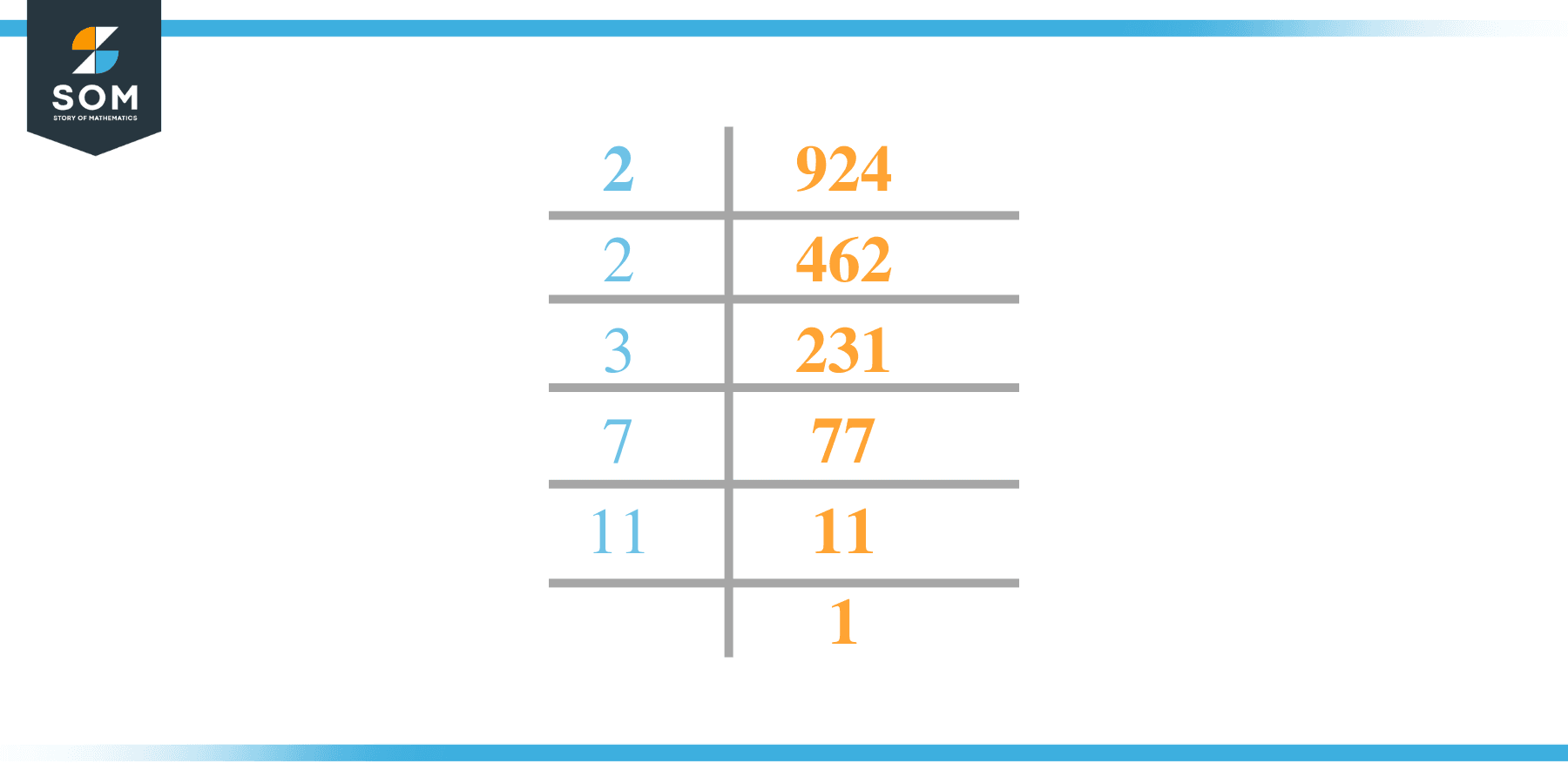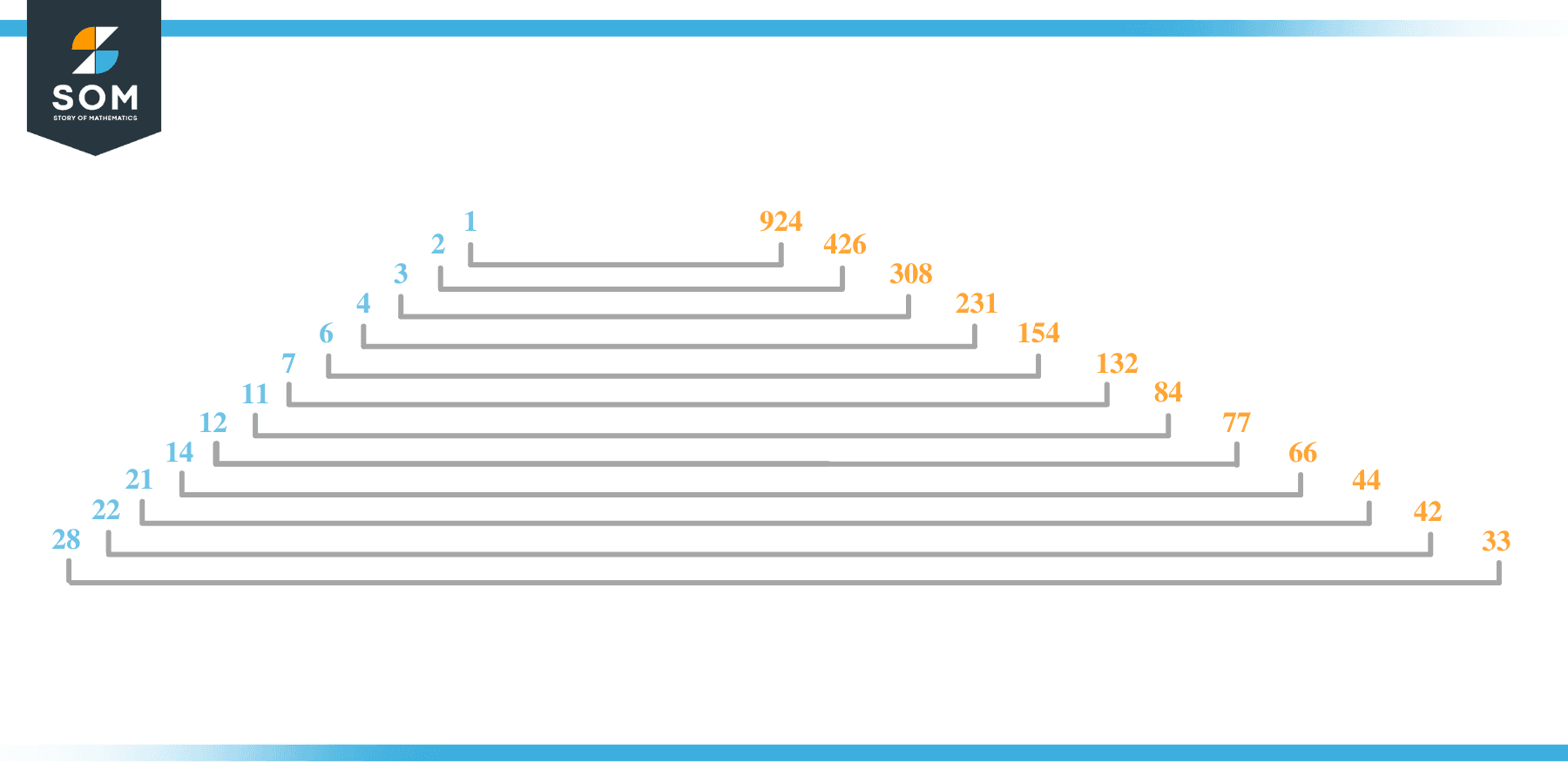# Factors of 924: Prime Factorization, Methods, and Examples

The factors of 924 are numbers that, when divided by 924, give zero as the remainder. The given number’s factors can be positive and negative.### Factors of 924

Here are the factors of number 924.

Factors of 924: 1, 2, 3, 4, 6, 7, 11, 12, 14, 21, 22, 28, 33, 42, 44, 66, 77, 84, 132, 154, 231, 308, 426, and 924.

### Negative Factors of 924

The negative factors of 924 are similar to its positive aspects, just with a negative sign.

Negative Factors of 924: –1, -2, -3, -4, -6, -7, -11, -12, -14, -21, -22, -28, -33, -42, -44, -66, -77, -84, -132, -154, -231, -308, -426, and -924

### Prime Factorization of 924

The prime factorization of 924 is the way of expressing its prime factors in the product form.

Prime Factorization: 2 x 2 x 3 x 7 x 11

In this article, we will learn about the factors of 924 and how to find them using various techniques such as upside-down division, prime factorization, and factor tree.

## What Are the Factors of 924?

The factors of 924 are 1, 2, 3, 4, 6, 7, 11, 12, 14, 21, 22, 28, 33, 42, 44, 66, 77, 84, 132, 154, 231, 308, 426, and 924. These numbers are the factors as they do not leave any remainder when divided by 924.

The factors of 924 are classified as prime numbers and composite numbers. The prime factors of the number 924 can be determined using the prime factorization technique.

## How To Find the Factors of 924?

You can find the factors of 924 by using the rules of divisibility. The divisibility rule states that any number, when divided by any other natural number, is said to be divisible by the number if the quotient is the whole number and the resulting remainder is zero.

To find the factors of 924, create a list containing the numbers that are exactly divisible by 924 with zero remainders. One important thing to note is that 1 and 924 are the 924’s factors as every natural number has 1 and the number itself as its factor.

1 is also called the universal factor of every number. The factors of 924 are determined as follows:

$\dfrac{924}{1} = 924$

$\dfrac{924}{2} = 426$

$\dfrac{924}{3} = 308$

$\dfrac{924}{4} = 231$

$\dfrac{924}{6} = 154$

$\dfrac{924}{7} = 132$

$\dfrac{924}{11} = 84$

$\dfrac{924}{12} = 77$

$\dfrac{924}{14} = 66$

$\dfrac{924}{21} = 44$

$\dfrac{924}{22} = 42$

$\dfrac{924}{28} = 33$

Therefore, 1, 2, 3, 4, 6, 7, 11, 12, 14, 21, 22, 28, 33, 42, 44, 66, 77, 84, 132, 154, 231, 308, 426, and 924 are the factors of 924.

### Total Number of Factors of 924

For 924, there are twenty four positive factors and twenty four negative ones. So in total, there are 48 factors of 924

To find the total number of factors of the given number, follow the procedure mentioned below:

1. Find the factorization/prime factorization of the given number.
2. Demonstrate the prime factorization of the number in the form of exponent form.
3. Add 1 to each of the exponents of the prime factor.
4. Now, multiply the resulting exponents together. This obtained product is equivalent to the total number of factors of the given number.

By following this procedure, the total number of factors of 924 is given as:

Factorization of 924 is 1 x 2$^2$ x 3 x 7 x 11.

The exponent of 1, 3, 7, and 11 is 1 where as 2 is 2.

Adding 1 to each and multiplying them together results in 48.

Therefore, the total number of factors of 924 is 48. Twenty four are positive, and twenty four factors are negative.

### Important Notes

Here are some essential points that must be considered while finding the factors of any given number:

• The factor of any given number must be a whole number.
• The factors of the number cannot be in the form of decimals or fractions.
• Factors can be positive as well as negative.
• Negative factors are the additive inverse of the positive factors of a given number.
• The factor of a number cannot be greater than that number.
• Every even number has 2 as its prime factor, the smallest prime factor.

## Factors of 924 by Prime FactorizationThe number 924 is a composite number. Prime factorization is a valuable technique for finding the number’s prime factors and expressing the number as the product of its prime factors.

Before finding the factors of 924 using prime factorization, let us find out what prime factors are. Prime factors are the factors of any given number that are only divisible by 1 and themselves.

To start the prime factorization of 924, start dividing by its most minor prime factor. First, determine that the given number is either even or odd. If it is an even number, then 2 will be the smallest prime factor.

Continue splitting the quotient obtained until 1 is received as the quotient. The prime factorization of 294 can be expressed as:

924 = 2 x 2 x 3 x 7 x 11

## Factors of 924 in PairsThe factor pairs are the duplet of numbers that, when multiplied together, result in the factorized number. Factor pairs can be more than one depending on the total number of factors given.

For 924, the factor pairs can be found as:

1 x 924 = 924

2 x 426 = 924

3 x 308 = 924

4 x 231 = 924

6 x 154 = 924

7 x 132 = 924

11 x 84 = 924

12 x 77 = 924

14 x 66 = 924

21 x 44 = 924

22 x 42 = 924

28 x 33 = 924

The possible factor pairs of 924 are given as (1, 924), (2, 426), (3, 308), (4, 231), (6, 154), (7, 132), (11, 84), (12, 77), (14, 66), (21, 44), (22, 42) and (28, 33).

All these numbers in pairs, when multiplied, give 924 as the product.

The negative factor pairs of 924 are given as:

1 x -924 = 924

-2 x -426 = 924

-3 x -308 = 924

-4 x -231 = 924

-6 x -154 = 924

-7 x -132 = 924

-11 x -84 = 924

-12 x -77 = 924

-14 x -66 = 924

-21 x -44 = 924

-22 x -42 = 924

-28 x -33 = 924

It is important to note that in negative factor pairs, the minus sign has been multiplied by the minus sign, due to which the resulting product is the original positive number. Therefore, 1, -2, -3, -4, -6, -7, -11, -12, -14, -21, -22, -28, -33, -42, -44, -66, -77, -84, -132, -154, -231, -308, -426, and -924 are called negative factors of X.

The list of all the factors of 924, including positive as well as negative numbers, is given below.

Factor list of 924: 1, -1, 2, -2, 3, -3, 4, -4, 6, -6, 7, -7, 11, -11, 12, -12, 14, -14, 21, -21, 22, -22, 28, -28, 33, -33, 42, -42, 44, -44, 66, -66, 77, -77, 84, -84, 132, -132, 154, -154, 231, -231, 308, -308, 426, -426, 924, and -924

## Factors of 924 Solved Examples

To better understand the concept of factors, let’s solve some examples.

### Example 1

How many factors of 924 are there?

### Solution

The total number of Factors of 924 is 48.

Factors of 924 are  1, 2, 3, 4, 6, 7, 11, 12, 14, 21, 22, 28, 33, 42, 44, 66, 77, 84, 132, 154, 231, 308, 426, and 924.

### Example 2

Find the factors of 924 using prime factorization.

### Solution

The prime factorization of 924 is given as:

924 $\div$ 2 = 426

426 $\div$ 2 = 231

231 $\div$ 3 = 77

77 $\div$ 7 = 11

11 $\div$ 11 = 1

So the prime factorization of 924 can be written as:

2 x 2 x 3 x 7 x 11 = 924